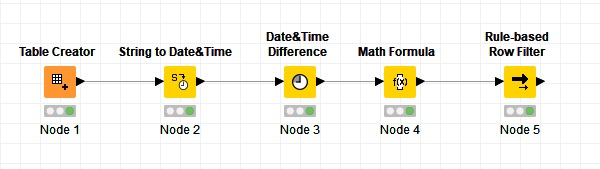# Exclude dates based on a set of rules

Hi there

I am trying to create a workflow that will exclude the first 6 days of data from a period of say two months. The criteria for the exclusion will test if the first 6 days have values that are less than the average value of the entire period. For example, I want to exclude if the daily vehicle volumes of the first 6 days are less than the average daily volume of the entire two months period.

So far I have tried using RowID to create a ordered list of dates then used multiple Rule-based Row Filter to exclude sequentially any one of the first 6 days of data if the daily volume meets the criteria of exclusion. I have not been successful in getting the results I want.

Any tips or help would be greatly appreciated.

Thanks

Hi,

You can use a “Date&Time Difference” node (after converting the date column) to calculate the days.
Then use a “Math Formula” to calculate the value column mean and at the end use a “Rule-based Row filter” node and input this rule: `\$value\$ < \$Mean\$ AND \$Days\$ <= 6 => TRUE` to exclude the values.

Best,
Armin6 days.knwf (23.4 KB)

1 Like

Hi Armin